# VLOOKUP

If you have known information on your spreadsheet, you can use `VLOOKUP` to search for related information by row. For example, if you want to buy an orange, you can use `VLOOKUP` to search for the price.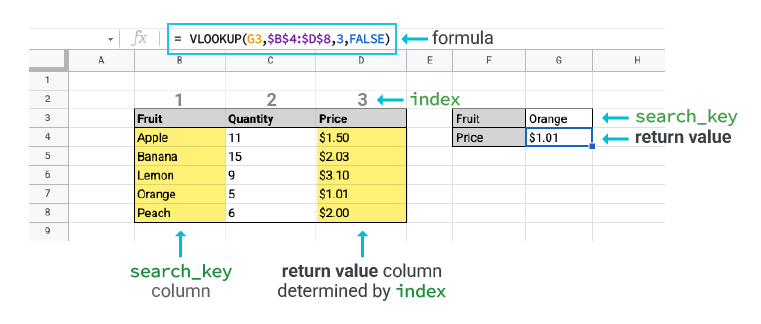### Syntax

=VLOOKUP(`search_key,` `range, index,` [`is_sorted`])

### Inputs

1. `search_key`: The value to search for in the first column of the range.
2. `range`: The upper and lower values to consider for the search.
3. `index`: The index of the column with the return value of the range. The index must be a positive integer.
4. `is_sorted`: Optional input. Choose an option:
• `FALSE` = Exact match. This is recommended.
• `TRUE` = Approximate match. This is the default if `is_sorted` is unspecified.
Important: Before you use an approximate match, sort your search key in ascending order. Otherwise, you may likely get a wrong return value. Learn why you may encounter a wrong return value.

### Return value

The first matched value from the selected `range`.
Technical details:
Example:
=VLOOKUP(G9, B4:D8, 3, FALSE)
=VLOOKUP("Apple", B4:D8, 3, TRUE)
 Inputs Description `search_key` This is the value you search in the first column of the `range`. If you expect a non-error value, the search key must be in the first column of the `range`. Cell reference is also supported. To do a simple check: If your `search_key` is located at B3, then your `range` should start with column B. `range` This is the `range` where: The function searches for the specified search key in its first column. `VLOOKUP` returns the value from the column specified by `index`. You can also use a named range. To return a non-error value, your search key must be in the first column of the `range`. To do a simple check: If your `search_key` is located at B3, then your `range` should start with column B. `index` Also called “Column number.” This is the index of the column in the `range` that contains the return value. The smallest possible index is 1. The largest possible index is the maximum number of columns in that `range`. After you set up the range, `VLOOKUP` only looks to the search key column, when index = 1 , or columns that are further right. Tip: When you use `VLOOKUP`, imagine that the columns of the `range` are numbered from left to right and start with 1. `is_sorted` This is an optional input. The two available choices are `TRUE` and `FALSE`. If `is_sorted` is `TRUE`, then `VLOOKUP` uses approximate match. Important: Before you use an approximate match, sort your search key in ascending order. Otherwise, you may get an unexpected value returned. Learn why you may encounter a wrong return value. If` is_sorted` is `FALSE`, then `VLOOKUP` uses exact match. If `is_sorted` is not specified, it is `TRUE` by default. We strongly recommend you: Use `FALSE` for `is_sorted` due to its consistent behavior whether or not the search key column is sorted. Always specify `is_sorted` for better readability, even though the input is optional.

 Outputs Description Return value This is the value that `VLOOKUP` returns based on your inputs. There’s only one return value from each `VLOOKUP `function. If there are multiple search key values that match, the value in the return value column whose associated search key is first matched in the search key column is returned. If #N/A is returned, a value isn’t found. If you encounter an expected value or error like #N/A or #VALUE!, begin to troubleshoot. If you want to replace #N/A with another value, learn more about how to use IFNA() on VLOOKUP().

## Basic VLOOKUP examples:

### VLOOKUP on different search keys

Use `VLOOKUP` to find the price of an Orange and Apple.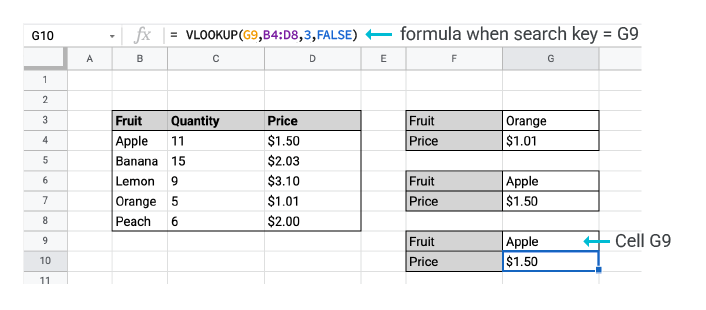Explanation:

When you use `VLOOKUP`, you can use different search keys such as "Apple" and "Orange."

To return a non-error value, these search keys must be in the first column of the `range`. If you don’t want to fill a value for search keys, you can also use a cell reference, for example "G9."
 `search_key` is "Orange" =VLOOKUP("Orange", B4:D8, 3, FALSE) Return value = \$1.01 `search_key` is "Apple" =VLOOKUP("Apple", B4:D8, 3, FALSE) Return value = \$1.50 `search_key` that uses cell reference of "Apple" in G9 =VLOOKUP(G9, B4:D8, 3, FALSE) Return value = \$1.50

### VLOOKUP on different column indexes

Use `VLOOKUP` to find the quantity of Oranges in the second index column.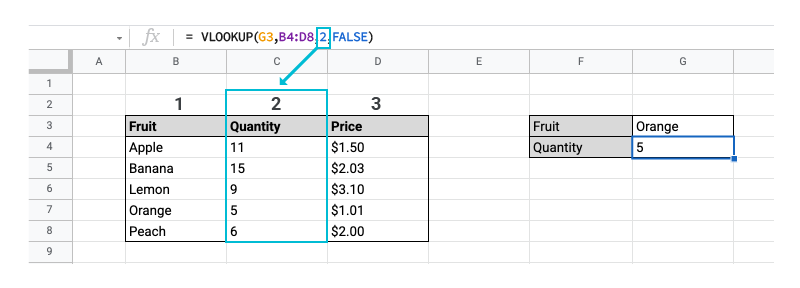Explanation:
When you use `VLOOKUP`, imagine that the columns of the `range` are numbered from left to right and start from 1. To find the target information, you must specify its column index. For example, column 2 for quantity.
 `Index` = 2 Find the quantity of oranges, which is the second column of the `range`. =VLOOKUP(G3, B4:D8, 2, FALSE) Return value = 5

### VLOOKUP exact match or approximate match

• Use `VLOOKUP` exact match to find an exact ID.
• Use `VLOOKUP` approximate match to find the approximate ID.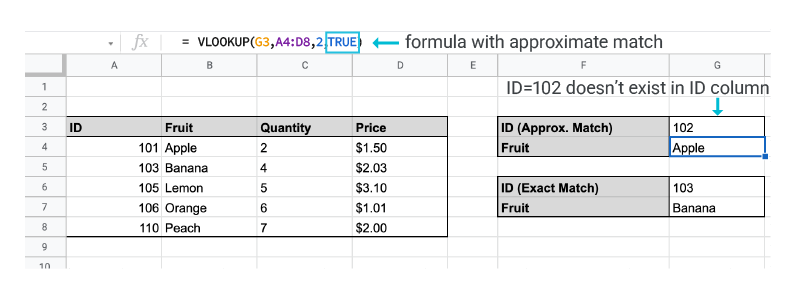Explanation:
Use an approximate match or `is_sorted` = `TRUE` when you search for a best match, but not an exact match.
If you want to search ID = 102, which doesn’t exist in the table, an approximate match takes one step back to give you ID = 101 as the result. This is because in the search key column, 101 is the closest value that is also less than 102.
An approximate match searches down the search key column until it finds a value that is larger than your search key. Then it stops on the row before the larger value and returns the value from the return value column on that row. That means if your search key column is not sorted in ascending order, you most likely get a wrong return value.
Important: Before you use an approximate match, sort your search key in ascending order to return the correct value. Otherwise, you may get an unexpected value returned.
When you search for the exact match, such as `is_sorted` = `FALSE`, it returns an exact match. For example, the fruit name for ID = 103 is "Banana." If there’s no exact match, you get a #N/A error. Due to its more predictable behavior, we recommend you use exact match.
 Exact match =VLOOKUP(G6, A4:D8, 2, FALSE) Return value = "Apple" Approximate match =VLOOKUP(G3, A4:D8, 2, TRUE) OR =VLOOKUP(G3, A4:D8, 2) Return value = "Banana"

## Common VLOOKUP applications

### Replace error value from VLOOKUP

You may want to replace an error value returned by `VLOOKUP` when your search key doesn’t exist. In this case, if you don’t want #N/A, you can use `IFNA()` functions to replace #N/A. Learn more about IFNA().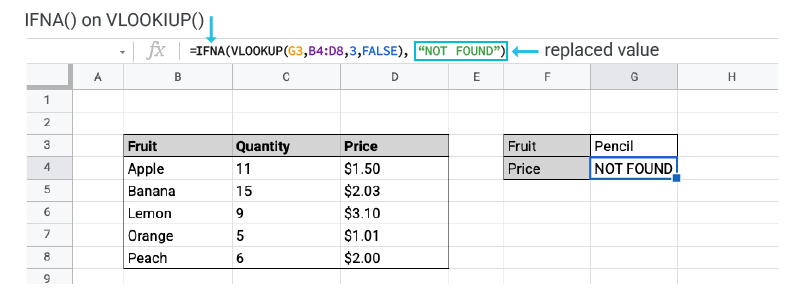Originally, `VLOOKUP` returns #N/A because the search key “Pencil” does not exist in the “Fruit” column. `IFNA()` replaces #N/A error with the second input specified in the function. In our case, it’s “NOT FOUND.” =IFNA(VLOOKUP(G3, B4:D8, 3, FALSE),"NOT FOUND") Return value = “NOT FOUND”

### VLOOKUP with multiple criteria

`VLOOKUP` can’t be directly applied on multiple criteria. Instead, create a new helper column to directly apply `VLOOKUP` on multiple criteria to combine multiple existing columns.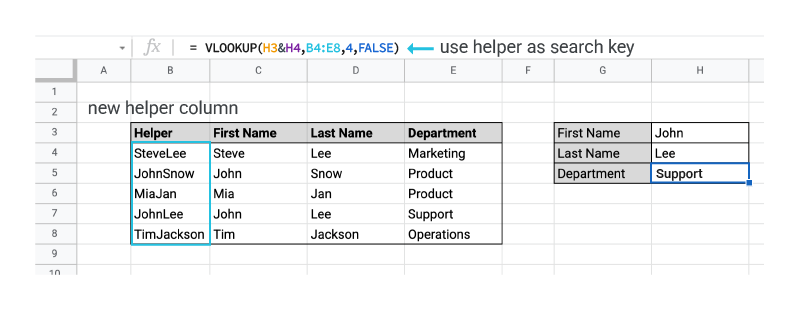1. You can create a Helper column if you use "&" to combine First Name and Last Name. =C4&D4 and drag it down from B4 to B8 gives you the Helper column. 2. Use cell reference B7, JohnLee, as the search key. =VLOOKUP(B7, B4:E8, 4, FALSE) Return value = "Support"

### VLOOKUP with wildcard or partial matches

In `VLOOKUP`, you can also use wildcards or partial matches. You can use these wildcard characters:
• A question mark "?" matches any single character.
• An asterisk "*" matches any sequence of characters.
To use wildcards in `VLOOKUP`, you must use an exact match: "`is_sorted` = `FALSE`".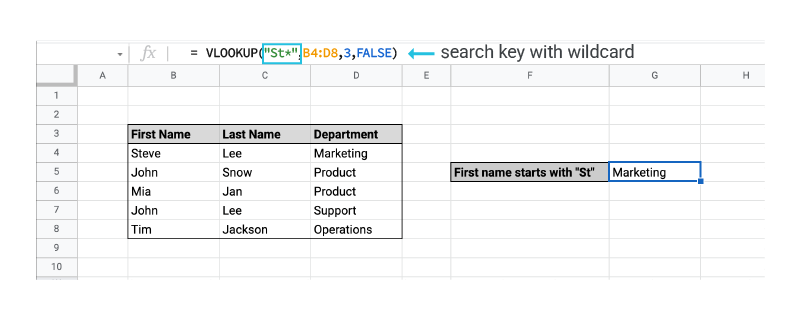"St*" is used to match anything that starts with "St" regardless of the number of characters, such as "Steve", "St1", "Stock", or "Steeeeeeve". =VLOOKUP("St*", B4:D8, 3, FALSE) Return value = "Marketing"

## Troubleshoot errors & best practices:

Wrong return value
• Returns an unexpected value: If you set `is_sorted` as `TRUE`, but your first column in the range isn’t sorted numerically or alphabetically in ascending order, then change is_sorted to `FALSE`.

• VLOOKUP gives the first match: `VLOOKUP` only returns the first match. If you have multiple matched search keys, a value is returned, but it may not be the expected value.
• Unclean data: Sometimes, values with spaces that trail and lead may seem similar but `VLOOKUP` treats them differently. For example, the following are different to `VLOOKUP`:
• " Apple"
• "Apple "
• "Apple"
To get your expected results, remove spaces before you use `VLOOKUP`.
#N/A
• If approximate or `is_sorted` = `TRUE` is used and if the search key in `VLOOKUP` is smaller than the smallest value in the first column, then `VLOOKUP` returns #N/A.
• If exact match or `is_sorted` = `FALSE` is used, then the exact match of the search key in `VLOOKUP` isn’t found in the first column. If you don’t want #N/A when the search key isn’t found in the first column, you can use the function IFNA().
#REF!
You might mistakenly specify a `range` with a number bigger than the maximum number of columns of the `range`. To avoid this, make sure you:
• Count the columns from the selected `range`, not the entire table.
• Start to count from 1 instead of 0.
#VALUE!
If you get #VALUE! error, you might have:
• Incorrectly input the text or the column name for the `index`.
• Entered a number smaller than 1 for the `index`. The `index `must be at least equal to 1 and smaller than the maximum number of columns of the `range`. `VLOOKUP` can only search in the search key column, when `index` = 1, or columns that are further right.

Important: `index` only accepts a number.

#NAME?
• You might have missed a quote in the search key when your `search_key` is text data.
Best practices

 To do Reason Use absolute references for `range` You should use: Absolute reference for `VLOOKUP` `range` VLOOKUP(G3, \$B\$3:\$D\$7, 3, FALSE) You should not use: VLOOKUP(G3, B3:D7, 3, FALSE) This prevents unpredictable changes in the `range` when it’s copied or dragged down. Sort the first column in ascending order when you use an approximate match, such as `is_sorted` = `TRUE`. If you use an approximate match or `is_sorted` = `TRUE`, you must sort the first column in ascending order. Otherwise, you most likely get a wrong return value. Learn more on how to sort. Clean your data before you use `VLOOKUP` Before you use `VLOOKUP`, remember to clean your data. Unclean data may cause `VLOOKUP` to return an unpredictable value. Here are some common pitfalls of unclean data: Spaces that lead: " apple" Spaces that trail: "apple " Blanks or spaces: "" and " " aren’t equivalent To trim white space that leads and trails, you can use Data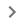Data cleanupTrim whitespace. Don't store number or date values as text Make sure your date or number values in the first column of your `VLOOKUP` range, such as the search key column, aren’t stored as text values. You may get an unexpected return value. On the top of Sheets, select your search key column. Tap Format MenuNumber. Choose an option depending on your desired data type: Date Number